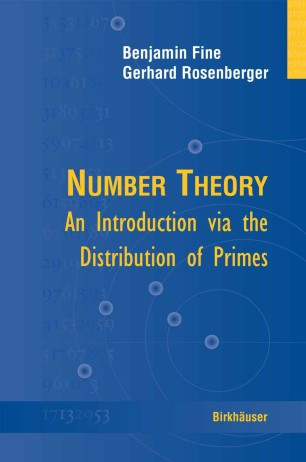# Number Theory

## An Introduction via the Distribution of Primes

• Benjamin Fine
• Gerhard RosenbergerTextbook

1. Front Matter
Pages i-xvi
2. Pages 1-5
3. Pages 7-54
4. Pages 55-131
5. Pages 133-196
6. Pages 197-251
7. Pages 253-331
8. Back Matter
Pages 333-342

### Introduction

This book provides an introduction and overview of number theory based on the distribution and properties of primes. This unique approach provides both a firm background in the standard material as well as an overview of the whole discipline. All the essential topics are covered: fundamental theorem of arithmetic, theory of congruences, quadratic reciprocity, arithmetic functions, and the distribution of primes.

Key Topics and Features:

* Solid introduction to analytic number theory, including full proofs of Dirichlet’s Theorem and the Prime Number Theorem

* Solid treatment of algebraic number theory, including a complete presentation of primes, prime factorizations in algebraic number fields, and unique factorization of ideals

* First treatment in book form of the AKS algorithm that shows that primality testing is of polynomial time

* Many interesting side topics, such as primality testing and cryptography, Fermat and Mersenne numbers, and Carmichael numbers

The book’s user-friendly style, historical context, and wide range of exercises from simple to quite difficult (with solutions and hints provided for select ones) make it ideal for self study as well as classroom use. Intended for upper level undergraduates and beginning graduate students, the only prerequisites are a basic knowledge of calculus, multivariable calculus, and some linear algebra. All necessary concepts from abstract algebra and complex analysis are introduced in the book.

### Keywords

Mersenne number Number theory Prime Prime number algorithms analysis cryptography linear algebra

#### Authors and affiliations

• Benjamin Fine
• 1
• Gerhard Rosenberger
• 2
1. 1.Department of MathematicsFairfield UniversityFairfieldUSA
2. 2.Fachbereich MathematikUniversität DortmundDortmundGermany

Industry Sectors